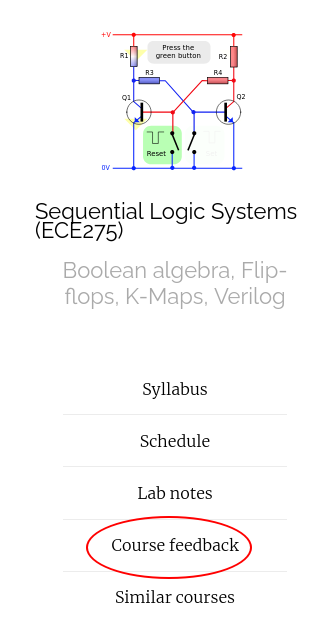## Announcments

• Midterm is on Oct 6th, 9 AM in class.

## Functional decomposition Ex4

$$\bA$$
$$\bB$$ $$B$$
$$\bC$$ $$C$$ $$\bC$$
$$\bD$$$$\bE$$ 0 1 1 1
$$E$$ 1 0 0 0
$$D$$ 0 1 1 1
$$\bE$$ 1 0 0 0
$$\bA$$
$$\bB$$ $$B$$
$$\bC$$ $$C$$ $$\bC$$
$$\bD$$$$\bE$$ 1 0 0 0
$$E$$ 0 1 0 0
$$D$$ 1 0 0 0
$$\bE$$ 0 1 0 0
Press b to see blackboard

## Place value number system

• Decimal: Base 10
• Binary: Base 2
 Decimal Binary 0 0 1 1 2 10 3 11 4 100 5 101 6 110 7 111 8 1000 9 1001 10 1010

## Value of a number

• A number system with base $$b$$ has at least $$b$$ unique symbols with special.
• A number system is has two operators $$+$$ and $$\cdot$$. The number system must be closed under the two operators.
• There is at least one symbol $$0$$ such that $$x + 0 = x$$
• There is at least one symbol $$1$$ such that $$x \cdot 1 = x$$
• A number in base $$b$$ written as $$d_n \dots d_1 d_0$$ has the value $d_n \dots d_1 d_0 = d_n b^n + \dots + d_1 b^1 + d_0 b^0$

 Decimal Hexadecimal 0 0 $$\vdots$$ $$\vdots$$ 9 9 10 A 11 B 12 C 13 D 14 E 15 F

## Arbitrarily new number system

Define two symbols $$0, 1$$ as required.
• ♥ = 0
• ♦ = 1

Define rest of the symbols in increments of $$♦$$
• ♣ = ♦ + 1
• ♠ = ♣ + 1

The value of ♠♦♥ = $$3\times 4^2 + 1 \times 4^1 + 0 \times 4^0$$

## Thanks, Questions, Feedback?

https://vikasdhiman.info/ECE275-Sequential-Logic/